# BACKGROUND

By the time I got the idea for what is now the pay-off method, the idea for a real option valuation method that uses fuzzy logic is something that I had been working on for about ten years. The work for me started around the change of the millennium, when I started to work for the Institute for Advanced Management Systems Research (IAMSR) that used to be a part of the Åbo Akademi university (but is a private company now) on a project called WAENO that concentrated on the valuation and profitability analysis of very large industrial real investments that we started to call “giga-investments”. The project results showed that the commonly used investment analysis methods have many weaknesses, especially when the investments are irreversible, take a long time to build, and have long economic lives. I finished my dissertation on the subject in 2004 (Download from RePEc). During and already before the project Christer Carlsson and Robert Fullér worked on a new formulation for the possibilistic mean value and variance of fuzzy numbers that were later on used in what is probably the first version of the Black-Scholes** formula that uses fuzzy numbers.

Today we can call the fuzzy Black-Scholes model a “hybrid”, as it uses both fuzzy and crisp numbers. During and after the WAENO project a number of systems were built, based on the hybrid Black-Scholes method and implemented in solving investment analysis problems identified by a number of industrial companies. The work of WAENO was continued by a research project called OptionsPorts. Others working within the projects on real options included Peter Majlender, Francisco Alcaraz Garcia, and Markku Heikkilä.

Unfortunately Peter passed away some time ago at the early age of 36. He is remembered as a very talented mathematician and as a very nice guy!

Since 2007 I started, after having spent about three years away from the academia in consulting, working again at the IAMSR for a research project called OptionsStrat. To my great disappointment I noticed that the usability, or rather the lack of usability, of real option valuation methods had practically remained at the level at which I had last seen it in 2004. In other words: at a level of non-usability for practitioners. This was a very big issue for the OptionsStrat project, as one of the goals of the project was the creation of a usable system for inexperienced users of real option valuation for the analysis and decision-support of R&D investments. Indeed going through the most commonly used option valuation methods with the practitioners was not something one really enjoyed, as the number of simplifications and (often intolerable) assumptions of reality made most of the meetings just an “academic exercise” – unfortunately soon forgotten by the practitioners. Fuzzy inputs, when shown graphically were however almost always very easily understood by the practitioners, as well as the ability of fuzzy numbers to show uncertainty and the reduction / increase in uncertainty (as information changes). Fuzzy NPV was also a no-brainer for most of the people I talked with.

The complexity of the Black-Scholes** model however usually made the method a black box for most (all) of the listeners. From my earlier experience in working with the giga-investments I knew that fuzzy numbers are highly usable, when uncertainty about the future is a major issue, and I knew that  what was needed was a method that would simplify the calculation of the real option value and profitability analysis as a whole. To tackle the problem I wanted to broaden my horizon on the issue and worked with Tuomas Multamäki on looking at the construct of the option valuation models and especially the derivation of standard deviation that is used in the models. I also read some very good books on the subject of randomness and our ability to forecast the future (N. Taleb) and a very interesting book on how Edward Thorp was the one who figured out a very nice way to value options before the Black-Scholes** formula was published in 1973.

** I want to point out the view of Haug & Taleb to what concerns using the the Black – Scholes model in the real world trading

# BIRTH OF THE METHOD

In the summer of 2008 I attended the 12th Annual International Conference on Real Options in Rio de Janeiro in Brazil. During that conference I attended a number of presentations that presented a number of works on the application of real options and the valuation of real options. The turning point of the conference for me was a presentation by Scott Mathews from Boeing Inc. where he presented and showed the application of what he called the Datar-Mathews method (for real option valuation). The Datar-Mathews method uses a simulation to generate a probability distribution of project outcomes from project cash-flow scenarios given by the responsible project managers – then the probability weighted mean value of the positive outcomes is calculated and multiplied by the probability of the positive outcomes (%) over all of the outcomes (100%) – the answer is real option value! Scott Mathews (and Vinay Datar) show that the answer from the method corresponds to the answer from the Black-Scholes** model under the same constraints (that are used in the Black – Scholes method).

After having seen and heard (and hopefully understood…) Scott Mathews presentation I started putting the pieces together and it became clear to me: the pay-off distribution of a project value that is calculated with fuzzy numbers is nothing more than the fuzzy NPV of the project. The mean value of the positive values of the fuzzy NPV is the possibilistic mean value of the positive fuzzy NPV values. And the real option value calculated from the fuzzy NPV is the possibilistic mean value of the positive fuzzy NPV values multiplied with the positive area of the fuzzy NPV over the total area of the fuzzy NPV. In other words, the real option value can be derived (without any simulation whatsoever) from the fuzzy NPV. These are the blocks that together make the (fuzzy) pay-off method for real option valuation.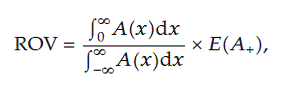Definition 1: fuzzy pay-off method for real option valuation. From the article “A Fuzzy Pay-Off Method for Real Option Valuation

To be totally clear here, simulation is not an absolutely necessary step in the Datar-Mathews method, so the two methods are not very different in that respect. But what is totally different is that the Datar-Mathews method is based on probability theory and as such has a very (fully) different foundation from the fuzzy pay-off method that is based on possibility theory. This means that the way the two models treat uncertainty is different.

What the difference means for the practitioner is increased simplicity and enhanced usability that translate to understandable real option valuation. I want to emphasize here that the work of Datar and Mathews has been a quantum leap as far as the understandability and simplifying real option valuation goes, but the pay-off method goes even further on that same path.

Naturally, a method is not really usable if it does not “bend” to the world of the most commonly used spreadsheet software and indeed that was the first task at hand for me also – what I did was a very simple file that calculated the real option value from a triangular fuzzy NPV (distribution) for the four different cases (whole NPV below zero, whole NPV above zero, number partially over zero, but peak above zero, number partially over zero, but peak below zero, [obviously including the zero at peak also]) by calculating the area of the positive part of the distribution by using triangles (graphically), just to test the method. Then together with Robert Fullér and József Mezei we finalized the mathematical formulation of the fuzzy pay-off method. In a published journal paper we illustrate the use of the method numerically and show solutions for the calculation of the fuzzy mean value of the positive side of the fuzzy NPV distributions for three different types of fuzzy numbers (triangular, trapezoidal, and an “ad-hoc” type fuzzy number). Later on I have sometimes thought that publishing the method in a not so great journal may not have been a very good idea, but it seemed to not matter at the time – what we did think about at the time was that the journal is open access.

An important issue to see here is: (real) option value comes from the pay-off distribution, not from the process that creates it, this means that when we are dealing with (real) options that do not have a gBm (geometric Brownian motion) type process behind them we need not use that process, but it is OK to use any process that we have identified to underlie, OR in case we are not able to identify a process we can estimate the distribution by, e.g., using cash-flow scenarios as a basis. The gBm is used in many models, because it returns a well defined pay-off distribution, not because it would be the best choice of process!

The good part here is that it is very common in companies to use cash-flow scenarios to model the future – this means that if a real option valuation model can use the data that is already available in companies AND does not force the company people to change the way they are doing things to fit the model, there will be much more interest in the model.

Furthermore, just using the scenarios to build the pay-off distribution and visually presenting it, even without the calculation of the real option value from the distribution is a MAJOR step forward in visualizing the risks involved in any investment.

# APPLICATION OF THE (FUZZY) PAY-OFF METHOD

Figure 1: calculating the real option value of an investment (click image to enlarge)In the picture above we can see on the first section in the top cash flow estimations for three investment cost scenarios (time in half-year blocks), the present value (PV) of the expected investment cash-flows is calculated with a 5% (risk free) discount rate. Below the cash flow table we can see the sum of the present values of the investment cash flow estimations cumulative for each of the future times (for each of the three scenarios). To the right (in yellow) we have the cumulative PV for the investment cash flows for years 0 to 10.

In the second section a table is presented for three scenarios of expected revenue cash flows, and the PV of these is calculated with a risk adjusted discount rate of 15% (revenue cash flows are risky); below these the cumulative PV of the revenue cash flows is presented.

To calculate the (expected) fuzzy net present value (FNPV) of the investment we deduct the cost from the revenue – this is done by deducting the PV of the lowest cost estimate from the PV of the highest revenue estimate, and the PV of the highest cost estimate from the PV of the lowest revenue estimate; to reach the maximum and the minimum values (the upper and lower values of the distribution). The base case (or the middle) scenario PV of costs is deducted from the PV of revenues to obtain the middle value of the distribution.

The three scenario NPVs are then used in the creation of the pay-off distribution, which in this case is triangular (because there are three scenarios). The pay-off distribution is constructed by:

(i)     observing that the best guess scenario is the most likely one and assigning it full degree membership in the set of expected outcomes;

(ii)    deciding that the maximum possible and the minimum possible scenarios are the upper and lower bounds of the distribution – there is also a simplifying assumption: to not consider values higher than the optimistic scenario and lower than the pessimistic scenario; and

(iii)   assuming that the shape of the pay-off distribution is triangular.

In other words:

We simplify reality and assign the highest degree of possibility (fully possible) to the “base” case (or the middle case) and the lowest (approaching zero) degree of possibility to the minimum and maximum values of the distribution. Thus the result is a triangular fuzzy distribution that we will use as the fuzzy NPV (distribution) for the investment = the pay-off distribution. In doing the above mentioned construction of the triangular fuzzy number we are simplifying reality. Other methods of constructing the fuzzy number exist, and the form of the fuzzy number may naturally be something else than triangular. Trapezoidal fuzzy numbers are also very easy to use and intuitive to understand. What is very good in building such a distribution is that it shows the size and shape of the uncertainty, that is, the uncertainty is showed as we see it and the placement of the peak of the triangular distribution shows where we think we will most likely find ourselves.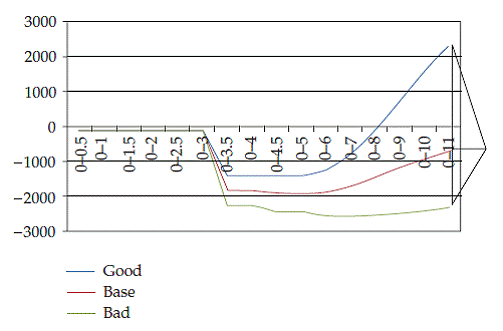Figure 2: cumulative NPV scenarios and how the pay-off distribution is formed

In the graphical presentation above we can see how the cumulative (distribution of the) NPV of the scenarios widens and the form of the scenarios resembles a cone. Indeed, what we have is very similar to an “inverted” cone of uncertainty.

# CALCULATION OF THE POSSIBILISTIC MEAN FOR THE POSITIVE PART OF A TRIANGULAR FUZZY PAY-OFF

First a bit about the notation:

a = corresponds to the best guess scenario NPV

α (alfa) = the distance between the minimum and the best guess scenario NPV

β (beta) = the distance between the maximum and the best guess scenario NPV

Keeping in mind the above definitions / clarifications we can now use the formulae below to calculate the possibilistic mean for the positive part of a triangular fuzzy pay-off.

1. When the whole distribution is “above zero”; when 0 < (a – α)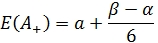2. When the distribution is partly “above zero” so that a is above zero, but a-α is below zero; when (a – α) < 0 < a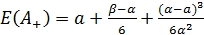3. When the distribution is partly “above zero” so that a is below zero, but a + β is above zero (a < 0 < a + β )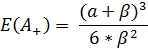4. When the distribution is totally “below zero”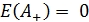Observe the following: In case 3 it is not ok to use the formula for case 1 by applying it to the “positive side triangle” separately. This would mean normalizing the positive side triangle (with a lower than 1.0 degree of membership) to a fuzzy number and causing the result to be wrong. In the case that you don’t understand what the above means, then just forget it! 🙂

By using the fuzzy pay-off method we can now calculate the real option value for the investment from the constructed fuzzy NPV distribution. We input the resulting E(A+) to the fuzzy pay-off method for real option valuation formula (also visible above in Definition 1) together with  ratio between the positive area of the pay-off distribution with the whole area.

# APPLICATION AND USE OF THE PAY-OFF METHOD

The fuzzy pay-off method for real option valuation has been integrated into a management information system in a large international industrial company for the valuation of research and development projects – they are using triangular fuzzy numbers. The method has been applied to many types of problems; valuation of patents, analysis of mergers and acquisitions, valuation of large industrial investments, and so on… many applications can be found from under the citing works tab.

The use of the pay-off method is taught within the larger framework of real options, for example at the Lappeenranta University of Technology and the Tampere University of Technology in Finland, and guest lectures have been given, for example, at the University of Trento (Italy) and the Palacky University in Olomouc, the Czech Republic.